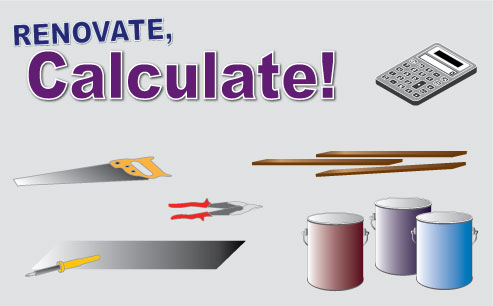# Renovate, Calculate!

Secondary KLA:
Mathematics
Educational levels:
Year 7, Year 8, Year 9, Year 10A student resource that explores the use of mathematics in the trades. Highly interactive investigations into ratio, areas of special quadrilaterals and right-angled trigonometry.

## NSW syllabus outcomes

(MA4-7NA) operates with ratios and rates, and explores their graphical representation

(MA4-13MG) uses formulas to calculate the areas of quadrilaterals and circles, and converts between units of area

(MA4-16MG) applies Pythagoras&#8217; theorem to calculate side lengths in right-angled triangles, and solves related problems

(MA5.1-10MG) applies trigonometry, given diagrams, to solve problems, including problems involving angles of elevation and depression

(MA5.2-13MG) applies trigonometry to solve problems, including problems involving bearings

## Australian curriculum content descriptions

(ACMNA173) Recognise and solve problems involving simple ratios

(ACMNA188) Solve a range of problems involving rates and ratios, with and without digital technologies

(ACMMG159) Establish the formulas for areas of rectangles, triangles and parallelograms and use these in problem solving

(ACMMG196) Find perimeters and areas of parallelograms, trapeziums, rhombuses and kites

(ACMMG222) Investigate Pythagoras’ Theorem and its application to solving simple problems involving right angled triangles

(ACMMG224) Apply trigonometry to solve right-angled triangle problems

Resource type:
Interactive Resource
ScOT topics:
Area, Trapeziums, Trigonometric Ratios, Ratios
File type:
text/html
Language/s:
en-AU
Author:
Centre for Learning Innovation
Publisher:
State of NSW, Department of Education
Date created:
Tuesday, 17 November 2009

Resource ID: 2bb8fd0c-1f62-4658-be9a-6fdae3817fd3